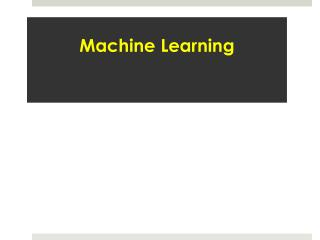# data science certification - PowerPoint PPT PresentationDownload Presentationdata science certification

data science certificationDownload Presentation## data science certification

- - - - - - - - - - - - - - - - - - - - - - - - - - - E N D - - - - - - - - - - - - - - - - - - - - - - - - - - -
##### Presentation Transcript

1. Machine Learning

2. Support Vector Machines

3. A Support Vector Machine (SVM) can be imagined as a surface that creates a boundary between points of data plotted in multidimensional that represent examples and their feature values The goal of a SVM is to create a flat boundary called a hyperplane, which divides the space to create fairly homogeneous partitions on either side SVMs can be adapted for use with nearly any type of learning task, including both classification and numeric prediction

4. Classification with hyper planes For example, the following figure depicts hyperplanes that separate groups of circles and squares in two and three dimensions. Because the circles and squares can be separated perfectly by the straight line or flat surface, they are said to be linearly separable

5. Which is the “best” Fit! In two dimensions, the task of the SVM algorithm is to identify a line that separates the two classes. As shown in the following figure, there is more than one choice of dividing line between the groups of circles and squares. How does the algorithm choose

6. Linear Hyperplane for 2-classes

7. SVM Objective OBJECTIVE: CONSTRAINT: Min

8. Nonlinearly separable data A cost value (denoted as C) is applied to all points that violate the constraints, and rather than finding the maximum margin, the algorithm attempts to minimize the total cost. We can therefore revise the optimization problem to:

9. Using kernels for non-linear spaces A key feature of SVMs is their ability to map the problem into a higher dimension space using a process known as the kernel trick. In doing so, a nonlinear relationship may suddenly appear to be quite linear. After the kernel trick has been applied, we look at the data through the lens of a new dimension: altitude. With the addition of this feature, the classes are now perfectly linearly separable

10. Neural Networks

11. Understanding neural networks An Artificial Neural Network (ANN) models the relationship between a set of input signals and an output signal using a model derived from our understanding of how a biological brain responds to stimuli from sensory inputs. Just as a brain uses a network of interconnected cells called neurons to create a massive parallel processor, ANN uses a network of artificial neurons or nodes to solve learning problems The human brain is made up of about 85 billion neurons, resulting in a network capable of representing a tremendous amount of knowledge For instance, a cat has roughly a billion neurons, a mouse has about 75 million neurons, and a cockroach has only about a million neurons. In contrast, many ANNs contain far fewer neurons, typically only several hundred, so we're in no danger of creating an artificial brain anytime in the near future

12. Biological to artificial neurons Incoming signals are received by the cell's dendrites through a biochemical process. The process allows the impulse to be weighted according to its relative importance or frequency. As the cell body begins accumulating the incoming signals, a threshold is reached at which the cell fires and the output signal is transmitted via an electrochemical process down the axon. At the axon's terminals, the electric signal is again processed as a chemical signal to be passed to the neighboring neurons.

13. This directed network diagram defines a relationship between the input signals received by the dendrites (x variables), and the output signal (y variable). Just as with the biological neuron, each dendrite's signal is weighted (w values) according to its importance. The input signals are summed by the cell body and the signal is passed on according to an activation function denoted by f A typical artificial neuron with n input dendrites can be represented by the formula that follows. The w weights allow each of the n inputs (denoted by xi) to contribute a greater or lesser amount to the sum of input signals. The net total is used by the activation function f(x), and the resulting signal, y(x), is the output axon

14. In biological sense, the activation function could be imagined as a process that involves summing the total input signal and determining whether it meets the firing threshold. If so, the neuron passes on the signal; otherwise, it does nothing. In ANN terms, this is known as a threshold activation function, as it results in an output signal only once a specified input threshold has been attained The following figure depicts a typical threshold function; in this case, the neuron fires when the sum of input signals is at least zero. Because its shape resembles a stair, it is sometimes called a unit step activation function

15. Network topology The ability of a neural network to learn is rooted in its topology, or the patterns and structures of interconnected neurons key characteristics • The number of layers • Whether information in the network is allowed to travel backward • The number of nodes within each layer of the network

16. Number of layers The input and output nodes are arranged in groups known as layers Input nodes process the incoming data exactly as it is received, the network has only one set of connection weights (labeled here as w1, w2, and w3). It is therefore termed a single-layer network

17. Thank you